Next: 5.4 Barrier Fluctuations Up: 5 Quantum Diffusion I: Previous: 5.2 Renormalization of the

# 5.3 Linear Coupling; One-Phonon Diffusion

In the case of a particle-phonon coupling linear in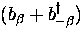we write the Hamiltonian of the excitations of the lattice plus the interaction between the lattice atoms and the interstitial muonium (which, in the adiabatic approximation is at the centre of the well, so this Hamiltonian depends on displacements of the lattice atoms only)

where the sum is over all phonons present. The coupling constant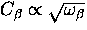governs the strength of the interaction with the interstitial. [54,53]

The effect of the polaron deformation and the origin of the associated trapping potential become apparent in calculating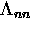.We start by using a normal oscillator shift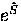in which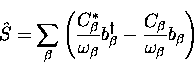(5)
to define new operators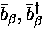,

Substituting these operators into the Hamiltonian, Eq. (5.9) becomes (noting that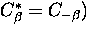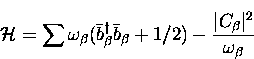(6)
which simply corresponds to a new set of harmonic oscillators with a shift in energy, but the one-phonon interaction has now been eliminated.

The wave functions we need to construct are then

We are now able to write the matrix elements of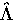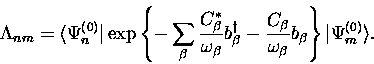(7)

The polaron effect corresponds to the diagonal elements,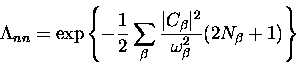(8)
which, with the number of phonons of frequency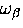now a function of temperature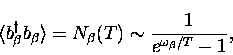(9)
reduces to (suppressing an overall constant)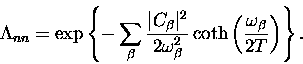(10)

It is usual in the literature to define the polaron exponent" as the sum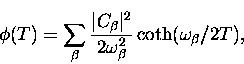(11)
so that the polaron effect manifests itself as a renormalization (reduction) of the raw tunnelling bandwidth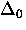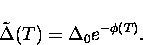(12)Next: 5.4 Barrier Fluctuations Up: 5 Quantum Diffusion I: Previous: 5.2 Renormalization of the